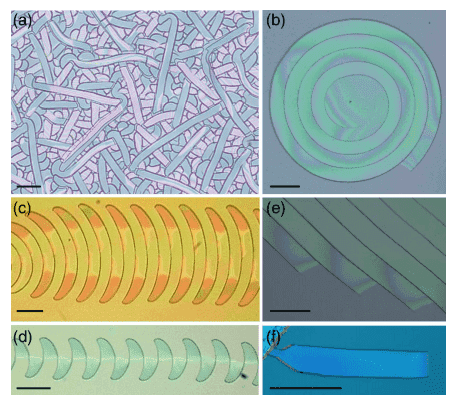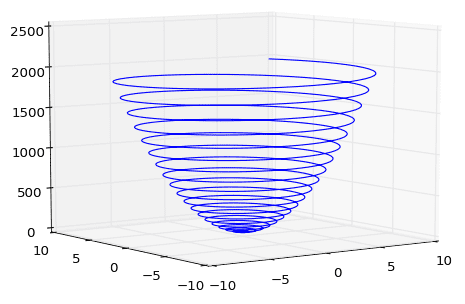# Use Lorentz Force to Find Magnetic Field Components

• I
So I am constructing an analogy between the self replicating fracturing effect on thin films and the path of a charged particle. (Qualitatively, several cracks have similar shapes to charged particle motion) I won't go into the details of the fracture mechanics, so I will only use E+M nomenclature.

My problem: Start with a parameterized path function, s, of a charged particle from which the velocity and acceleration functions can be easily derived. (a and R are constants)
$$\vec{s} = ( tcos(at) , tsin(at),(x^{2} + y^{2}) R )$$
I want to use this in a modified Lorentz Force equation where the mass-charge ratio is set to one (m/q=1)In the equation, I ultimately want to find a way to solve for all x, y, z components of E and B without resorting to Maxwell's equations.
$$dv/dt = E + v × B$$

Current attempt: In order to reduce the amount of unknowns from 6 to 3, (i.e. from Ex, Ey, Ez, Bx, By, Bz to just Bx, By, Bz) I thought I can use the relation between kinetic energy and the electric field. I am not sure if this is the right thing to do, so feel free to suggest a different method for minimizing unknowns. I used the following relation for this (m/q=1 again):
$$Work = qEs = \frac{1}{2}mv^{2}$$

So now s, v, dv/dt, and each component of E is known thus leaving the components of B left to be solved. The following vector equation is the result of taking the cross product:
$$(a_{x},a_y,a_z )= (E_x,E_y,E_z)+(v_yB_z-v_zB_y,v_zB_x-v_xB_z, v_xB_y-v_yB_x)$$

I think this then simplifies to:
$$\begin{pmatrix} 0& -v_{z} &v_{y} \\ v_{z}&0 &-v_{x} \\ -v_{y}& v_{x}& 0 \end{pmatrix}\begin{pmatrix} B_{x}\\ B_{y}\\ B_{z} \end{pmatrix} =\begin{pmatrix} a_{x}-E_{x}\\a_{y}-E_{y} \\ a_{z}-E_{z} \end{pmatrix}$$
Which doesn't seem to have a solution. What identities can I implement to yield solutions for this equation? Do I need to set one of the B components to 0? Is my equation for E useful in this case? Can I solve for Ex, Ey, Ez, Bx, By, Bz numerically- what numerical method would be useful?

tldr: I just want to know how to take a particle path and derive the electric and magnetic field components

Feel free to ask any questions for clarification!

Twigg
Gold Member
If your interest is in thin film cracking, can't you reduce the problem to 2 spatial dimensions? This would be the same as adding the constraints ##v_{z} = 0## and ##\frac{dv_{z}}{dt} = 0##, which means ##E_{z} + (v_{x}B_{y} - v_{y}B_{x}) = 0##. That would take you down to five unknowns.

To simplify the problem (whether you work in 2D or 3D), you will have to introduce the constraint of a steady-state field: ##\nabla \times \vec{E} = 0##, and of course ##\nabla \cdot \vec{B} = 0##. This will get incredibly messy, because it couples the equations of motion of nearby points.

One thing you could try: write an implicit finite-difference algorithm for solving the field equations over a grid of points, then further constrain this by adding the equations of motion given the velocity and acceleration at each point, and see if that gives you a fully defined system of equations. It's not exactly easy or guaranteed to work (the equations of motion might give you an under-defined system, it's hard to say).

Example in 2D:

In 2D, you only have ##E_{x}, E_{y}##, and ##B_{z}## to worry about, and ##\nabla \cdot \vec{B} = 0## doesn't matter. Suppose I take a grid of 4 points with spacing a, where (1,1) is the bottom left, (1,2) is the bottom right, (2,1) is the top left, (2,2) is the top right.

The field equations at (1,1) say ##\frac{E_{y}(1,2) - E_{y}(1,1)}{a} - \frac{E_{x}(2,1) - E_{x}(1,1)}{a} = 0##. This is one equation in twelve unknowns (3 unknown field variables per point, and 4 points).

The equations of motion at (1,1) say ##\vec{a} = \vec{E} + \vec{v} \times \vec{B}##, where ##\vec{a}## and ##\vec{v}## are known constants. This is 2 equations in 12 unknowns.

Taking both field equations and equations of motion together, the point (1,1) gives you 3 equations in 12 unknowns. If you repeat this process for the three remaining points in the grid, you obtain a total of 12 equations in 12 unknowns. Assuming the equations are all linearly independent, you will have a unique solution for the fields. You could check this by writing a 12x12 linear system for all these equations and taking its determinant. If it is zero, the method doesn't work. If it isn't zero, you can use any algorithm for solving linear systems to find the E and B fields.

Thanks, do you have any insight for the 3D example? Can it be done using the same algorithm you proposed for 2D? I am working in 3D because the delamination of the thin film cracks creates an upwards peeling effect along with the planar propagation of the crack.

So, the example I used for the path function was the Archimedian spiral (b), but it actually has a parabaloid shape. When I started this work, I was thinking in 2D, but the delamination peeling is actually really integral to these cracks. (The peeling is seen via the color warping between crack lines)Twigg
Gold Member
A paraboloid shape simplifies things, because that means the force in the z-axis is a constant. Since ##z = Rt^{2}##, you know that ##a_{z} = 2R## and thus ##F_{z} = 2mR##. A constant ##F_{z}## requires that ##B_{x} = B_{y} = 0## and ##F_{z} = qE_{z}##, or else the z-component of acceleration would depend on velocity in the plane. ##\nabla \cdot \vec{B} = 0## implies that ##B_{z}## is constant. That makes the problem effectively 2D, and maybe the algorithm I suggested can solve it.

In general, I suppose you could extend the algorithm I suggested to 3D, in which case the points would have indices, and you would have to use all four of the static field equations at each point plus the three equations of motion per point. Try a 2x2x2 example by hand to see if you get enough equations to fully determine the fields.

If it's not too much to ask, do you think you can run through the 3D example?

Twigg
Gold Member
Sure thing! Sorry for not elucidating.

Consider a 2 by 2 by 2 cubic grid of points, where each point is given by three indices as shown in the attached image (sorry for the low quality!). You have 6 unknowns per point and 8 points, so 48 unknowns total.

First, we have to formulate the field equations over the discrete mesh. In general, if we use (i,j,k) to denote the indices of the points, then ##\nabla \times \vec{E}=0## turns into three finite-difference equations (using forward differences only):

##\frac{E_{z}(i,j+1,k) - E_{z}(i,j,k)}{\Delta y} - \frac{E_{y}(i,j,k+1)-E_{y}(i,j,k)}{\Delta z} = 0##
##\frac{E_{x}(i,j,k+1) - E_{x}(i,j,k)}{\Delta z} - \frac{E_{z}(i+1,j,k)-E_{z}(i,j,k)}{\Delta x} = 0##
##\frac{E_{y}(i+1,j,k) - E_{y}(i,j,k)}{\Delta x} - \frac{E_{x}(i,j+1,k)-E_{x}(i,j,k)}{\Delta y} = 0##

(It might work out better if you use the average of forward and backward differences for the discrete derivative, but those are practical details you'll only know once you implement. See https://en.wikipedia.org/wiki/Finite_difference for explanation of forward and backward differences.)

And the remaining field equation ##\nabla \cdot \vec{B} = 0## becomes

##\frac{B_{x}(i+1,j,k) - B_{x}(i,j,k)}{\Delta x} + \frac{B_{y}(i,j+1,k)-B_{y}(i,j,k)}{\Delta y} + \frac{B_{z}(i,j,k+1) - B_{z}(i,j,k)}{\Delta z} = 0 ##

This gives 4 field equations per point and 8 points, so 32 equations in 48 unknowns.

Then apply the equations of motion at each point, ##\vec{a}(i,j,k) = \vec{E}(i,j,k) + \vec{v}(i,j,k) \times \vec{B}(i,j,k)## where ##\vec{a}## and ##\vec{v}## are known for all (i,j,k). That gives 3 equations per points and 8 points, so 24 equations in 48 unknowns.

Taking the field equations and equations of motion together, you have 56 equations in 48 unknowns. My interpretation of this is that not every velocity/acceleration pattern is compatible with a static electromagnetic field. There is a catch though, because if you do this numerically the odds of getting a perfectly solvable system are slim, because all it takes is one slight deviation in the last decimal place of a floating point number to make the system unsolvable. With that in mind, you may need to resort to a least-squares solution if the direct approach fails.

Let me know if anything wasn't clear.

P.S.: As you can see, the linear systems get very large very fast (in this case, 56 equations in 48 variables for an 8 point grid). You will need to use sparse matrix encoding (supported in MATLAB, BLAS, Lapack, and Armadillo, that I know of, plus probably a lot of others I don't know of) to reduce the memory load.

#### Attachments

Last edited:
Ok, so I have attempted your method, but I just learned forward/backward difference methods last semester. I have reduced the problem significantly and felt an update was finally appropriate.

For the spiral-paraboloid shape $$(atcos(at),atsin(at),Rt^{2})$$
I can make 2 assumptions:
1.) The B and E-fields are parallel and are only in the z direction (pointing out the mouth of the paraboloid).
2.) Since the acceleration in the z direction is just 2R (constant), the E-field is also constant- denoted E0

So this really helps reduce the Lorentz Equation $$\vec{a} = \vec{v}_{y}\vec{B}_{z}\hat{x}+\vec{v}_{x}\vec{B}_{z}\hat{y}+E_{0}\hat{z}$$
Where the acceleration and velocity vector functions are known. Can Bz be solved in this case? I'm sorry if my questions seems rudimentary; I tried solving and got two different values for Bz. I'm not sure if I trust my methods though. I tried the following...
$$\vec{v}_{y}\vec{B}_{z}=\frac{\delta v_{x}}{\delta{t}} , \vec{v}_{x}\vec{B}_{z} =-\frac{\delta v_{y}}{\delta{t}}$$